# 周长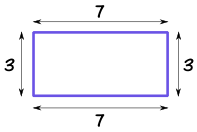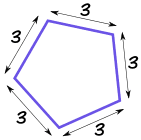圆的周长叫圆周： 圆周 = 2π × 半径## 周长公式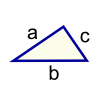三角形 周长 = a + b + c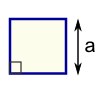正方形 周长 = 4 × a a = 边长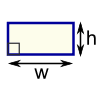矩形 周长 = 2 × (w + h) w = 宽 h = 高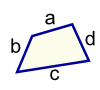四边形 周长 = a + b + c + d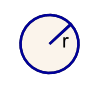圆 圆周 = 2πr r = 半径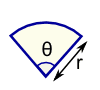扇形 周长 = r(θ+2) r = 半径 θ = 角度（单位为弧度）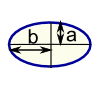椭圆 周长 = 非常困难！